# 基于视频的海上运动目标入侵检测Video Based Intrusion Detection for Moving Targets at Sea

DOI: 10.12677/JISP.2020.92012, PDF, HTML, XML, 下载: 163  浏览: 940  科研立项经费支持

Abstract: Our country’s existing maritime security protection facilities are a little behind in terms of ob-servation, monitoring and vigilance measures. Therefore, on the basis of using Faster R-cnn to identify ship types, this paper proposes an intrusion detection method for moving targets at sea without air and flow, and analyzes the influence of air and flow on this method. At present, this method can identify the ship as an aircraft carrier, other warships and civilian ships, and auto-matically alarm when the target enters the warning area.

1. 引言

2. 海上运动目标入侵检测方法的设计

1) 绊线绘制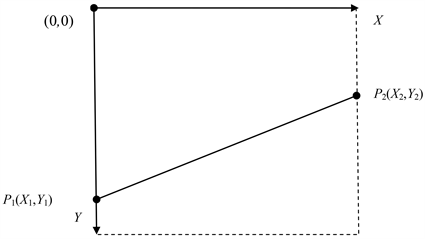Figure 1. Schematic diagram of trip line drawing

2) 海上运动目标检测

3) 入侵检测判定Figure 2. Tripping line diagram

$\begin{array}{c}\left(\stackrel{\to }{{\text{AP}}_{1}}×\stackrel{\to }{{\text{AP}}_{2}}\right)\ast \left(\stackrel{\to }{{\text{CP}}_{1}}×\stackrel{\to }{{\text{CP}}_{2}}\right)=|\left(\stackrel{\to }{{\text{AP}}_{1}}×\stackrel{\to }{{\text{AP}}_{2}}\right)|\ast |\left(\stackrel{\to }{{\text{CP}}_{1}}×\stackrel{\to }{{\text{CP}}_{2}}\right)|\ast \mathrm{cos}180\\ =-|\left(\stackrel{\to }{{\text{AP}}_{1}}×\stackrel{\to }{{\text{AP}}_{2}}\right)|\ast |\left(\stackrel{\to }{{\text{CP}}_{1}}×\stackrel{\to }{{\text{CP}}_{2}}\right)|<0\end{array}$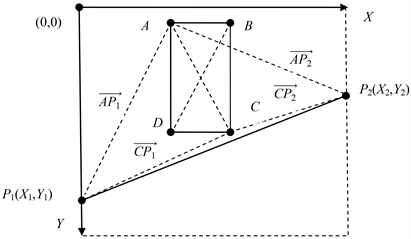Figure 3. Schematic diagram of non-tripwire

$\begin{array}{c}\left(\stackrel{\to }{{\text{AP}}_{1}}×\stackrel{\to }{{\text{AP}}_{2}}\right)\ast \left(\stackrel{\to }{{\text{CP}}_{1}}×\stackrel{\to }{{\text{CP}}_{2}}\right)=|\left(\stackrel{\to }{{\text{AP}}_{1}}×\stackrel{\to }{{\text{AP}}_{2}}\right)|\ast |\left(\stackrel{\to }{{\text{CP}}_{1}}×\stackrel{\to }{{\text{CP}}_{2}}\right)|\ast \mathrm{cos}0\\ =|\left(\stackrel{\to }{{\text{AP}}_{1}}×\stackrel{\to }{{\text{AP}}_{2}}\right)|\ast |\left(\stackrel{\to }{{\text{CP}}_{1}}×\stackrel{\to }{{\text{CP}}_{2}}\right)|>0\end{array}$

4) 报警

3. 风流对海上运动目标入侵检测的影响

1) 横摇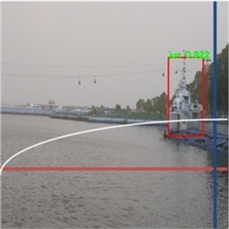(a)(b)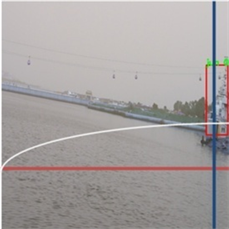(c)

Figure 4. Schematic diagram of the impact of rolling motion on intrusion detection

2) 纵摇(a)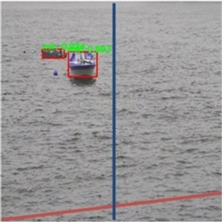(b)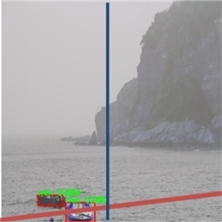(c)

Figure 5. Schematic diagram of the impact of pitch motion on intrusion detection

3) 垂荡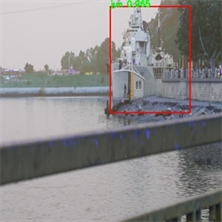(a)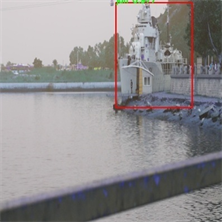(b)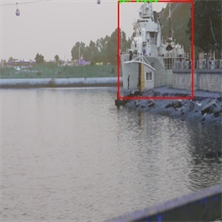(c)

Figure 6. Schematic diagram of the influence of heave motion on intrusion detectionTable 1. Influence of air flow on intrusion detection of moving targets at sea

4. 实验结果及分析Table 2. Intrusion detection results of moving targets at sea

4.1. 摄像机固定在陆地上监视近岸海域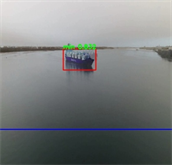(a) 初始帧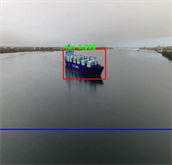(b) 第100帧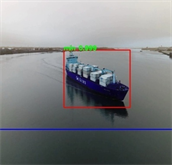(c) 第200帧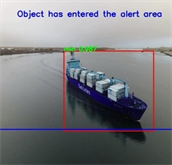(d) 第238帧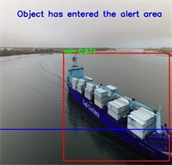(e) 第258帧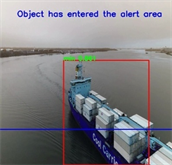(f) 第316帧

Figure 7. The camera fixed on land to monitor the offshore sea areas

4.2. 无人艇或舰船携带摄像机监视远距离海域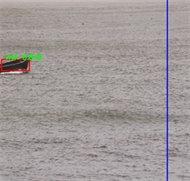(a) 初始帧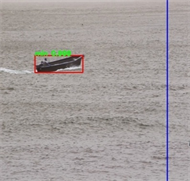(b) 第50帧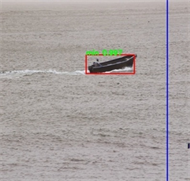(c) 第100帧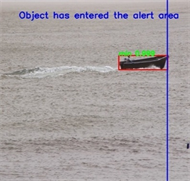(d) 第133帧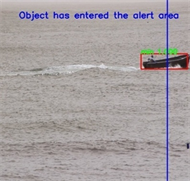(e) 第163帧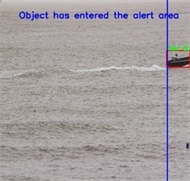(f) 第183帧

Figure 8. The camera fixed on ships or unmanned ships to monitor remote sea areas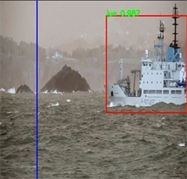(a) 初始帧b) 第100帧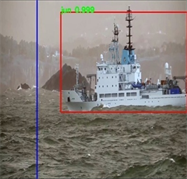(c) 第200帧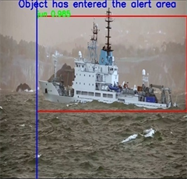(d) 第284帧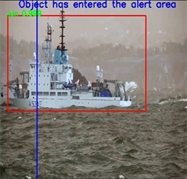(e) 第484帧(f) 第678帧

Figure 9. The camera fixed on ships or unmanned ships to monitor remote sea areas

4.3. 无人艇或舰艇的避碰报警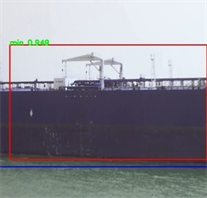(a) 初始帧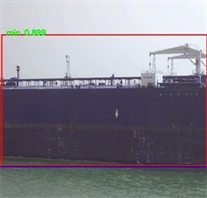(b) 第30帧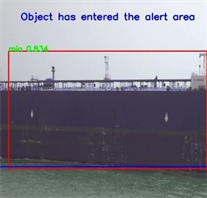(c) 第60帧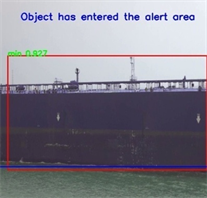(d) 第94帧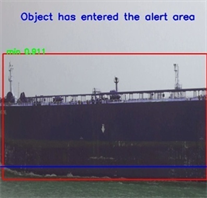(e) 第124帧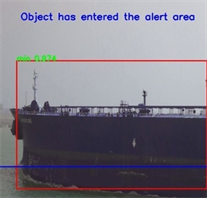(f) 第158帧

Figure 10. Collision avoidance alarm of unmanned craft or vessel

5. 结束语

  张明杰, 李翠华, 刘明业, 张龙飞. 基于多背景模型的海面运动目标检测[J]. 北京理工大学学报, 2005(6): 495-498 + 508.  陈慧敏. 近岸海域运动目标检测与跟踪算法的设计与实现[D]: [硕士学位论文]. 成都: 电子科技大学, 2013.  李祎承, 胡钊政, 初秀民. 基于图像处理的内河船舶目标提取与特征值计算[J]. 交通信息与安全, 2015, 33(3): 1-8.  郭石磊, 饶进军. 基于动态背景下的海上运动目标检测[J]. 计量与测试技术, 2016, 43(12): 3-5 + 8.  马忠丽, 文杰, 梁秀梅, 陈虹丽, 赵新华. 无人艇视觉系统多类水面目标特征提取与识别[J]. 西安交通大学学报, 2014, 48(8): 60-66.  赵亮, 王晓峰, 袁逸涛. 基于深度卷积神经网络的船舶识别方法研究[J]. 舰船科学技术, 2016, 38(15): 119-123.  戚超, 王晓峰. 基于卷积神经网络的运输船舶分类识别方法[J]. 微型机与应用, 2017, 36(17): 52-55.